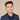# How To Convert String Date To Date In Swift

To convert a string date to a date object in Swift, you should use `DateFormatter()`.

## Convert ISO8601 To Date In Swift

``````import Foundation
let isoDate = "2020-01-22T11:22:00+0000"
let dateFormatter = DateFormatter()
dateFormatter.locale = Locale(identifier: "en_US_POSIX")
dateFormatter.dateFormat = "yyyy-MM-dd'T'HH:mm:ssZ"
if let date = dateFormatter.date(from: isoDate) {
// do something with date...
}``````

## Convert Month Day Year String To Date In Swift

Say you have a string in the format of “January 20, 2020”

Here’s how you would convert that date.

``````import Foundation
let dateString = "January 20, 2020"
let dateFormatter = DateFormatter()
dateFormatter.locale = Locale(identifier: "en_US_POSIX")
dateFormatter.dateFormat = "MMMM d, yyyy"
if let date = dateFormatter.date(from: dateString) {
// do something with date...
}``````

To convert a date where they use the short form of months such as “Jan 20, 2020” you can replace the `MMMM` with just `MMM`:

``````import Foundation
let dateString = "Jan 20, 2020"
let dateFormatter = DateFormatter()
dateFormatter.locale = Locale(identifier: "en_US_POSIX")
dateFormatter.dateFormat = "MMM d, yyyy"
if let date = dateFormatter.date(from: dateString) {
// do something with date...
}``````

## Convert Day Of Week String To Date In Swift

If you have a day of week in the string such as a “Monday” or “Friday” here’s how you do it:

``````import Foundation
let dateString = "Monday, Jan 20, 2020"
let dateFormatter = DateFormatter()
dateFormatter.locale = Locale(identifier: "en_US_POSIX")
dateFormatter.dateFormat = "EEEE, MMM d, yyyy"
if let date = dateFormatter.date(from: dateString) {
// do something with date...
}``````

If the string has a short-form of the day such as “Mon” you can use `EEE` instead:

``````import Foundation
let dateString = "Mon, Jan 20, 2020"
let dateFormatter = DateFormatter()
dateFormatter.locale = Locale(identifier: "en_US_POSIX")
dateFormatter.dateFormat = "EEE, MMM d, yyyy"
if let date = dateFormatter.date(from: dateString) {
// do something with date...
}``````

## Convert Number Date String To Date In Swift

### US Numbered Date Format

Typically in the US, the format is MM/DD/YYYY.

To convert this format to a date object use the following code:

``````import Foundation
let dateString = "01/20/2020"
let dateFormatter = DateFormatter()
dateFormatter.locale = Locale(identifier: "en_US_POSIX")
dateFormatter.dateFormat = "MM/dd/yyyy"
if let date = dateFormatter.date(from: dateString) {
// do something with date...
print(date)
}``````

### International Numbered Date Format

A typical format used internationally is DD-MM-YYYY.

To convert this string to a date object use this code:

``````import Foundation
let dateString = "20-01-2020"
let dateFormatter = DateFormatter()
dateFormatter.locale = Locale(identifier: "en_US_POSIX")
dateFormatter.dateFormat = "dd-MM-yyyy"
if let date = dateFormatter.date(from: dateString) {
// do something with date...
print(date)
}``````

## Convert Epoch or Unix Date To Date In Swift

To convert a unix or epoch date to a date object in Swift use this code:

``let date = Date(timeIntervalSince1970: timeResult)``
If you liked this post and want to learn more, check out The Complete iOS Developer Bootcamp. Speed up your learning curve - hundreds of students have already joined. Thanks for reading!## Eddy Chung

I teach iOS development on ZeroToAppStore.com.